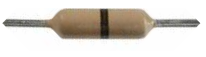﻿ Zero-ohm Resistors# Zero-ohm ResistorsA zero-ohm resistor is a resistor that has almost no resistance at all; its resistance is near 0 ohms.

A zero-ohm resistor can be wire wound or it can be surface mount. For surface mount resistors, there wil be a '0' on it indicating it is a zero-ohm resistor. You can see this on the image below. For wire wound resistors, there will be a single black color band around the body of the resistor. You can see this on the image above.Zero-ohm resistors are available in 1/8W and 1/4W power ratings. Of course, zero-ohm resistors aren't perfectly "resistance-less". Due to the fact that it is made up of material shows it has some internal resistance. The actual resistance, though, is very small. The actual resistance of a 1/8W zero-ohm resistor is about 0.004Ω, whereas a 1/4W zero-ohm resistor has a resistance of approximately 0.003Ω.

### Why Use Zero-ohm Resistors?

The question you probably have is, why are zero-ohm resistors even used? Why not just use a regular jumper wire, since the resistor carries negligent resistance?

The reason zero-ohm resistors are used is because components in most printed circuit boards are inserted by automatic insertion machines rather than manually by humans. In some instances, it may be necessary to short two points on the printed circuit board, in which case a wire would normally be placed between the two points. However, these automatic insertion machines can only handle components such as resistors, not jumper wires. A separate jumper wire machine would have to be used to insert jumper wires or the jumper wires had to be installed manually by a person. So, instead zero-ohm resistors are used in place.

Zero-ohm reisstors are also ideal in that they can be more easily removed than jumper wires. So, if any after-the-fact design changes needed to be made, the zero-ohm resistor could be removed and a new component be put in its place.

Note: If you are simply breadboarding and hobbying with electronics, chances are you won't need to use or be acquanited with zero-ohm resistors; you simply would just use jumper wires for these purposes. However, if you get more serious, and you want to turn the breadboard prototype into a professional printed circuit board, those jumper wires may turn into zero-ohm resistor usage.